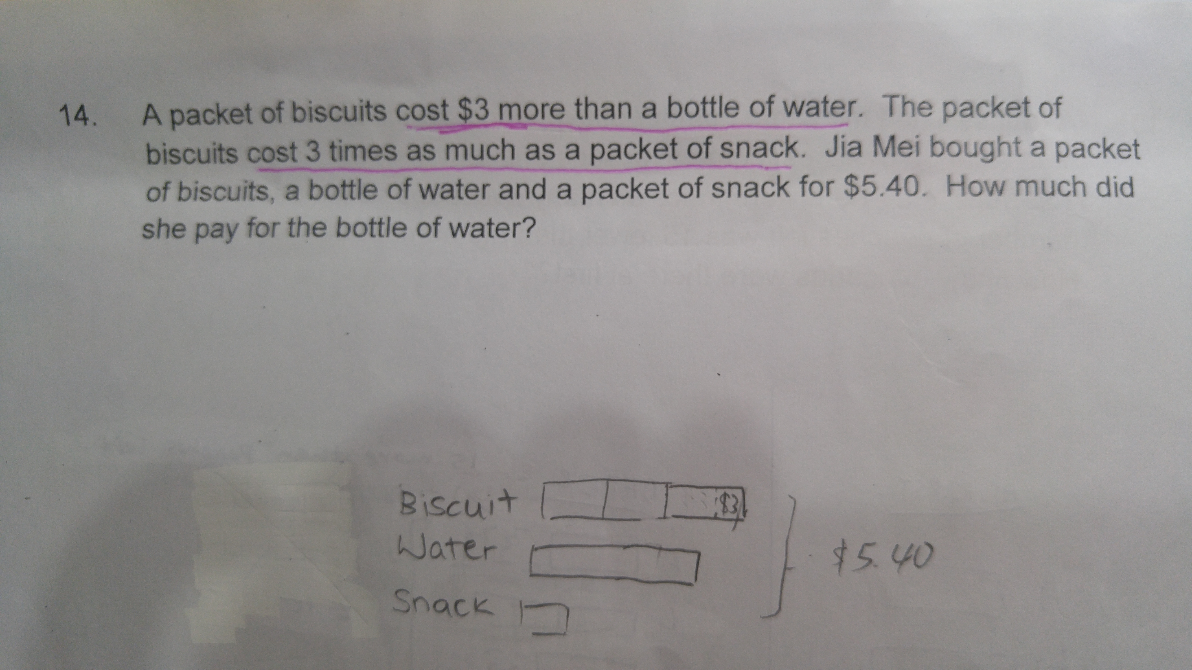# QuestionThis is a Part Whole question.

 Snack Biscuit water Total Quantity 1u 3u 3u – 3 7u – 3

7u – 3 = 5.40

7u = 5.40 + 3 = 8.40

1u = 8.40 ÷ 7 = 1.20

3u = 3 × 1.20 = 3.60

3.60 – 3 = \$0.60

0 Replies 0 Likes ✔Accepted Answer